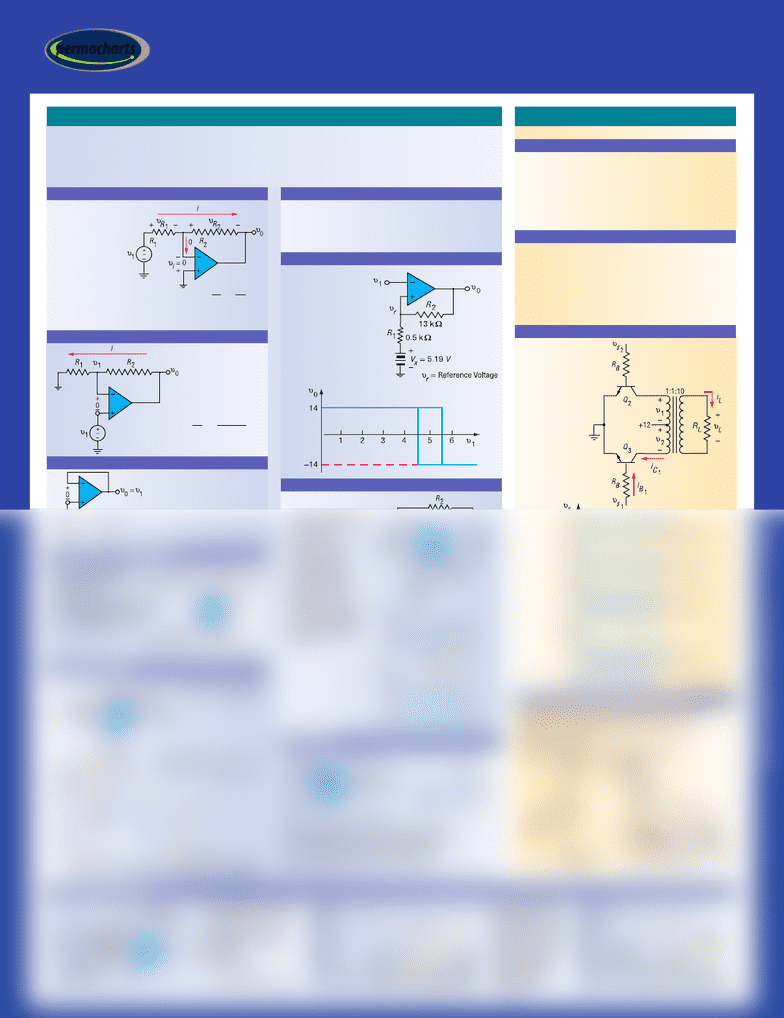Get 2 days of unlimited access
Class Notes (1,000,000)
US (420,000)
UA (100)
4400 (5)
All (5)
Reference Guide

# Permachart - Marketing Reference Guide: Schmitt Trigger, Square Wave, Negative Feedback

Department
Electrical Engineering
Course Code
4400:307
Professor
All
Chapter
Permachart

This preview shows half of the first page. to view the full 2 pages of the document.permacharts.com
TM
permacharts
Electronics II
• Linear applications involve negative feedback (amplifier,
summer DAC and ADC, integrators, differentiators, filters, etc.)
As a result, the voltage at its input is held at ª 0
• Non-linear applications include comparators, Schmitt triggers,
oscillators, ideal diodes, etc.
These involve positive or no feedback
In these cases, the high gain of the op amp causes the output
to become saturated at either its positive or negative extreme,
about a volt short of the supply voltages
(+) and (–) at the input
indicate the polarity of vi
that makes v0positive
vi(–) is inverting input
terminal because if
vi(), then v0Ø
vi(+) indicates
non-inverting input terminal
because if vi(+), then v0Ø
• Gain depends only on R1and R2
• Ideally, vi= 0, A= , Rin = , Rout = 0
INVERTING CONFIGURATION
V
V
R
R
0
1
2
1
=
υ
υ
0
1
12
1
=+
RR
R
uo= u1
• Only purpose is buffering
NON-INVERTING CONFIGURATION
VOLTAGE FOLLOWER
• If u1is grounded, then
u2sees an inverting
configuration
• If u2is grounded, then u1sees
a non-inverting configuration
DIFFERENTIAL CONFIGURATION
υυυ
02112
=
()( )
RR
INTEGRATOR CIRCUIT
υυ
0
1
1
11
= =
∫∫
C
idt
CR
dt
ν
νπ
0
11 1
1
1
2
===
Z
RfCR
c
R1dominates impedance
of capacitor at f> 5.0 kHz
• Therefore, ccan be
neglected so ideal gain is
flat R2/R1
without R
iCddt
iR R Cd dt
1
1
0221
:
=
==
υ
υυ
• If 2 curves cross at a
steep angle, phase
margin goes to 0 and
circuit will be unstable
• Therefore, response
of op amp must be
made to go flat
(horizontal) in area
of crossing
For real op amps, there is a small DC input offset voltage VIO
and a small DC input bias current IB
The effect of each is DC offset at the output
General purpose op amp has typical VIO = 5 mV
dc , IB= 0.1 mA
DC OFFSET
• An op amp alone can be
used as comparator but
it can chatter
Solution: Schmitt trigger
can provide hysteresis by
positive feedback
• Input terminals compare
two voltages and output
is either +14 or –14,
depending on which
input voltage is higher
SCHMITT TRIGGER
• Schmitt trigger can be
modified to make an
oscillator to generate a
square wave
• As with Schmitt
trigger, urkeeps
changing due to
positive feedback
• Negative feedback
produces u1by
charging a capacitor
• Frequency depends on
the value of the RC
product
OSCILLATOR
• Circuit realizing a nearly ideal diode characteristic
• For upositive, the circuit is a voltage follower
• For unegative, the output is an open circuit
IDEAL DIODE
DIFFERENTIATOR CIRCUIT
Operational Amplifiers (OP AMPS)
Where signal at base (gate) determines if switch is on or off
“Off” when iB= 0 iE= 0 is the cutoff state
VBE £0.3 V
• If VBE is made more negative than ª–5 V, then the
base-emitter junction may break down
“On” when iB iC/ bﬁu
CE is 0.2 V: saturation state
uBE is still 0.7 V, as in the active state
Modeled by a resistance rds(on) in the “on” state
• Control is by gate-source voltage
VPtypically –10 V to –1 V
rds(on) typically 30 Wto 500 W
“Off” : uGS £VP;On” : uGS = 0
VFET, DFET, and HEXFET have rds(on) of only a few ohms
Example: Converts
power at 12 V
dc to power
at 118 V
ac
BIPOLAR
FIELD-EFFECT TRANSISTOR (FET)
• Used when transistor cannot handle voltage or current
• SCRs can be rated as high as 2500 V and 1500 A
Turned on by a gate
Turns off only when voltage across it reverses
POWER INVERTER USING EMITTER-COUPLED LOGIC
SILICON CONTROLLED RECTIFIERS (SCR)
Transistors As Switches
###### You're Reading a Preview

Unlock to view full version